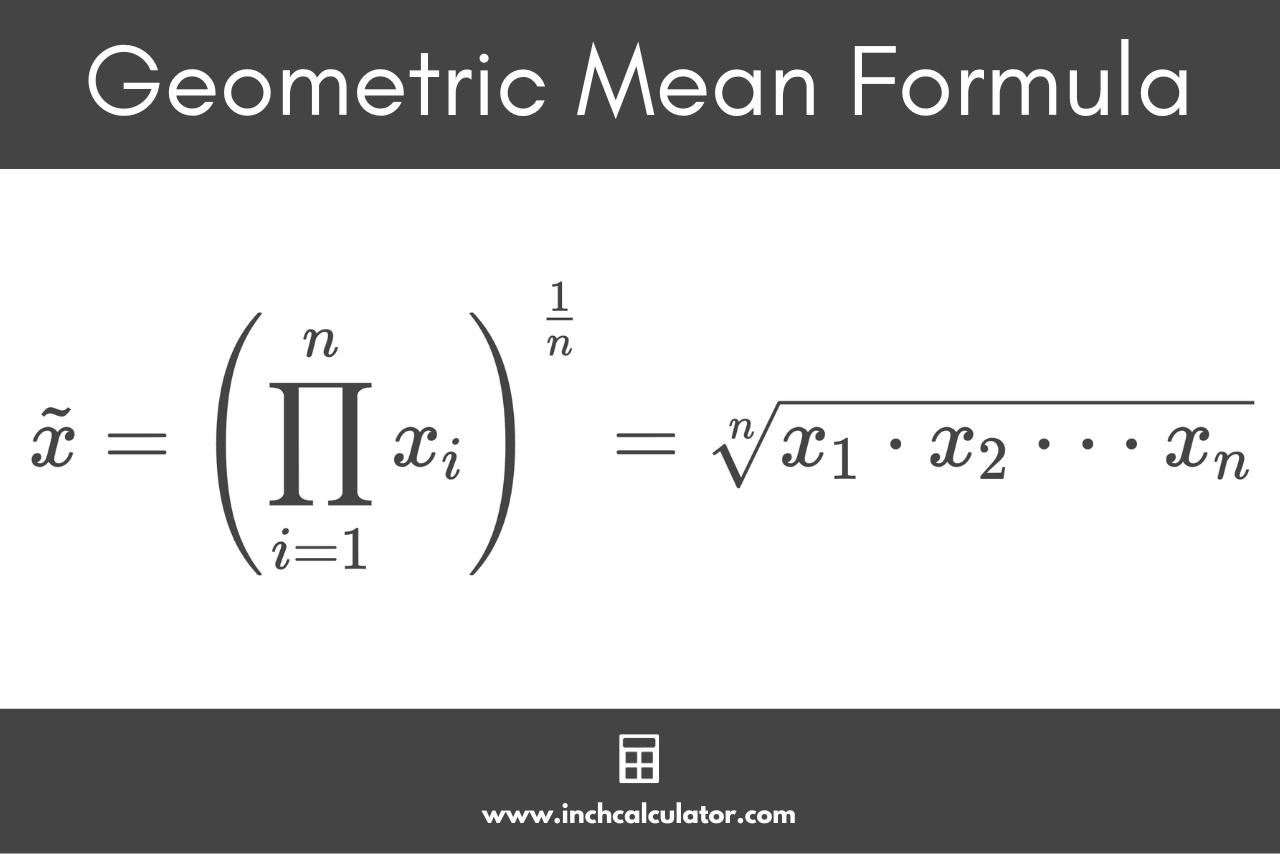# Geometric Mean Calculator

Find the geometric mean of a data set by entering the numbers in the dataset below.

Separate numbers using a comma (,)

## Results:

### Steps to Solve

#### Apply the Geometric Mean Formula

\tilde{x} = \sqrt[n]{x_{1} \cdot x_{2} \cdot\cdot\cdot x_{n}}

#### Step One: Find the Product of the Numbers in the Set

x_{1} \cdot x_{2} \cdot\cdot\cdot x_{n}

#### Step Two: Find the nth Root

\tilde{x} = \sqrt[n]{\text{product}}
Learn how we calculated this below

## How to Calculate the Geometric Mean

In statistics, given a dataset of n numbers, the geometric mean is the average of a data set that is found using the nth root of the product of each number in the set.

The geometric mean, sometimes also called the geometric average, is similar to the arithmetic mean in that it is a measure of the central tendency of the data, but instead of measuring the center using addition and division, it uses multiplication and the root.

The geometric mean is a way of measuring the average of a progression of data, which is why it’s often used in finance, investing, and economics, for instance to calculate compound interest or return on investment.

### Geometric Mean Formula

The geometric mean can be expressed using the following formula:

\tilde{x} = \left ( \prod_{i=1}^{n}x_{i} \right )^{\frac{1}{n}}

Where:
xi = each number in the set
n = total number of values in the set

The formula states that the geometric mean is equal to the product of a set of n numbers, raised to the power of 1 divided by n.

This formula is equivalent to the nth root of the product of the numbers in the set, so the simplified formula to find the geometric mean formula is:

\tilde{x} = \sqrt[n]{x_{1} \cdot x_{2} \cdot\cdot\cdot x_{n}}

So, the geometric mean is equal to the nth root of the product of each number xi in the set, where n is equal to the number of elements in the set.Using the formula, you can find the geometric mean in a few steps.

### Step One: Find the Product

First, find the product of all of the numbers in the set. You can do this by multiplying each number in the set together.

### Step Two: Find the Root

Then, count the number of items in the set and set this value to n. Once you have the count, take the nth root of the product.

For example: let’s find the geometric mean for the numbers [2,3,5,7]

Let’s start by finding the product of all of the numbers.

2 \cdot 3 \cdot 5 \cdot 7 = 210

Then, let’s take the nth root of 210, with n being equal to 4, since there are four numbers in the set.

\tilde{x}_{g} = \sqrt{210}
\tilde{x}_{g} = 3.806754

So, the geometric mean of the data set above is roughly equal to 3.806754.

Note that all values in the data set must be greater than zero for the geometric mean formula to work correctly; negative numbers are not supported by the formula. Using negative numbers could result in taking the square root of a negative, resulting in an imaginary number.